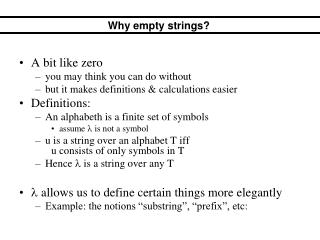DownloadDownload PresentationWhy empty strings?

Why empty strings?

Télécharger la présentationWhy empty strings?

- - - - - - - - - - - - - - - - - - - - - - - - - - - E N D - - - - - - - - - - - - - - - - - - - - - - - - - - -
Presentation Transcript

1. A bit like zero you may think you can do without but it makes definitions & calculations easier Definitions: An alphabeth is a finite set of symbols assume  is not a symbol u is a string over an alphabet T iff u consists of only symbols in T Hence  is a string over any T  allows us to define certain things more elegantly Example: the notions “substring”, “prefix”, etc: Why empty strings?

2. Definitions (from first lecture) • A string u is a substring of w if there exist other • strings x & y s.t. w = xuy. • bab is a substring of babba. • If u is a substring of w, and x above is , then u • is a prefix of w. • If uw, then u is a proper prefix. • ba is a prefix of babba. • If u is a substring of w, and y above is , then u • is a suffix of w. • If uw, then u is a proper suffix. • bba is a suffix of babba.

3. for any string u, u=u=u it follows that === ... some properties

4. for any string u, u=u=u it follows that === ... some properties

5. We could easily have defined FSAs in such a way that  edges don’t exist Yet, our definition allowed FSAs to contain  edges We did this by allowing such moves explicitly:  edges

6. FSA: Formal Definition • A Finite State Automaton (FSA) is a 5-tuple (Q, I, F, T, E) where: • Q = states = a finite set; • I = initial states = a nonempty subset of Q; • F = final states = a subset of Q; • T = an alphabet; • E = edges = a subset of Q (T + ) Q. FSA = labelled, directed graph = set of nodes (some final/initial) + directed arcs (arrows) between nodes + each arc has a label from the alphabet. a Example: formal definition of A1 Q = {1, 2, 3, 4} I = {1} F = {4} T = {a, b} E = { (1,a,2), (1,b,4), (2,a,3), (2,b,4), (3,a,3), (3,b,3), (4,a,2), (4,b,4) } a,b 2 3 a b 1 a A1 b 4 b

7. Recall a string is accepted by an FSA iff the string is the label of a path from initial to final node Example: this NDFSA accepts the string 01 and the string 001. After reading the first 0, the FSA can either read a 0 or a λ implications for strings accepted by FSA  1 j k f i 0 0 1 m

8. Sometimes we want to prove things like “Every so-and-so language can be accepted by an FSA” One often proves such things by showing how FSAs can actually be constructed The resulting FSAs often contain  edges Example: proof that every Regular Expression has an equivalent FSA. usefulness of  edges in FSAa